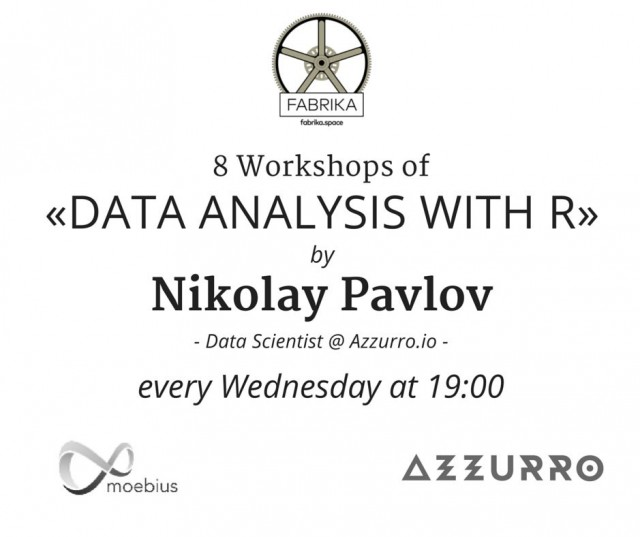Home Business Kharkivens will be able to visit 8 free IT workshops

# Kharkivens will be able to visit 8 free IT workshopsStarting from March 30, every Wednesday, Nikolay Pavlov, Data Scientist @ Azzurro.io, will hold a series of Data Analysis with R workshops, reports the IP-portal DOU.UA.

Data Analysis with R includes eight 2-hour sessions dedicated to Data Science.

Participants of the workshops will be able to learn about functional programming (Scala) and machine learning (R&Spark) absolutely free.

Organizer of training – Kharkiv Computer Science Group and the informal association of R&D companies and individual researchers.

Curriculum plan:

Introduction to data
R programming language
Observations and variables
Relationship between variables
Population and sample
Dependent and independent variables
Experimental design and sampling methods

Data exploration, visualization and cleaning
Data import, cleaning and manipulations
Scatter plot
Histogram, mean, variance and standard deviation
Box plots, quartiles, median and outliers
Data transformations
Categorical data, contingency tables and bar plot

Probability
Outcome, random process and Law of Large numbers
Independence
Conditional, marginal and joint probabilities
Multiplication Rule
Bayes theorem
Random variables, Expected Value, Variance
Probability distributions: PDF, CDF
Normal distribution
Geometric distribution
Binomial distribution

Statistical Inference
Point estimates
Confidence interval
Hypothesis testing
Type I, type II errors, power
Paired data, different of two means
T-distribution
Inference for categorical data

Regression analysis
Linear regression and least squares (LS)
Conditions for fitting regression line
Residuals analysis, R^2
Interpretation and inference
Multiple regression
Model selection
Logistic regression

Predictive Analytics
Machine learning and Supervised learning
Regression / Classification
Error functions
Linear model
Decision Trees, Random Forest, Neural Networks, SVM
Cross-validation
Hyperparameters tuning

BigData, R and Apache Spark
Resilient Distributed Datasets (RDD)
Map-Reduce
SparkR, Data Frame operations
Machine Learning in Spark

Where: Fabrika.space (Blagoveshchenska Street, 1).
When: every Wednesday from 30 March to 18 May
Time: 19:00 to 21:00